# How to Calculate and Solve for Performance Index | Material Selection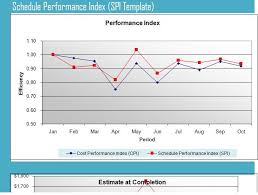The image above represents performance index.

To compute for performance index, two essential parameters are needed and these parameters are Shear Stress at Fracture (τf) and Density (ρ).

The formula for calculating the performance index:

P = τf2/3 / ρ

Where:

P = Performance Index
τf = Shear Stress at Fracture
ρ = Density

Let’s solve an example;/
Find the performance index when the shear stress at fracture is 32 and the density is 10.

This implies that;

τf = Shear Stress at Fracture = 32
ρ = Density = 10

P = τf2/3 / ρ
P = 322/3/10
P = 10.079 / 10
P = 1.0079

Therefore, the performance index is 1.0079.

Nickzom Calculator – The Calculator Encyclopedia is capable of calculating the performance index.

To get the answer and workings of the performance index using the Nickzom Calculator – The Calculator Encyclopedia. First, you need to obtain the app.

You can get this app via any of these means:

To get access to the professional version via web, you need to register and subscribe for NGN 2,000 per annum to have utter access to all functionalities.
You can also try the demo version via https://www.nickzom.org/calculator

Apple (Paid) – https://itunes.apple.com/us/app/nickzom-calculator/id1331162702?mt=8
Once, you have obtained the calculator encyclopedia app, proceed to the Calculator Map, then click on Materials and Metallurgical under Engineering.Now, Click on Material Selection under Materials and Metallurgical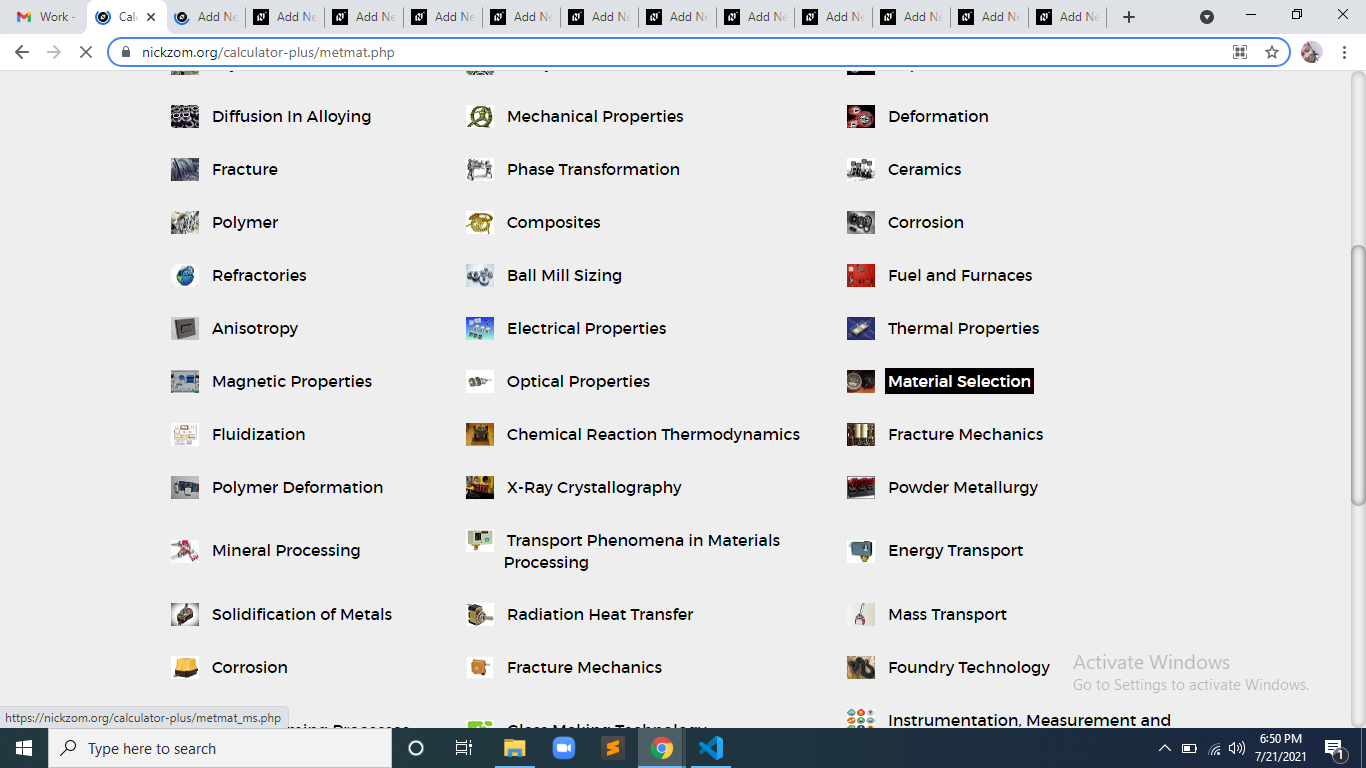Now, Click on Performance Index under Material Selection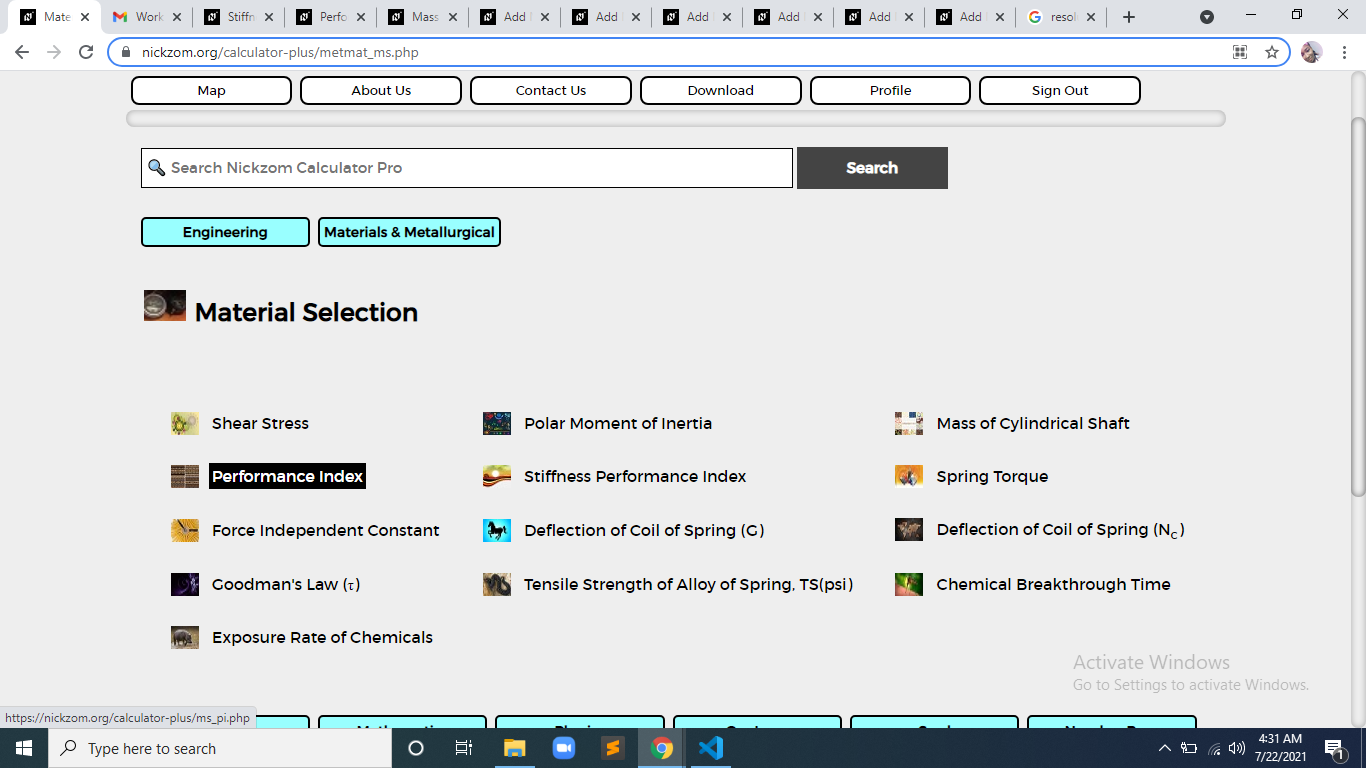The screenshot below displays the page or activity to enter your values, to get the answer for the performance index according to the respective parameter which is the Shear Stress at Fracture (τf) and Density (ρ).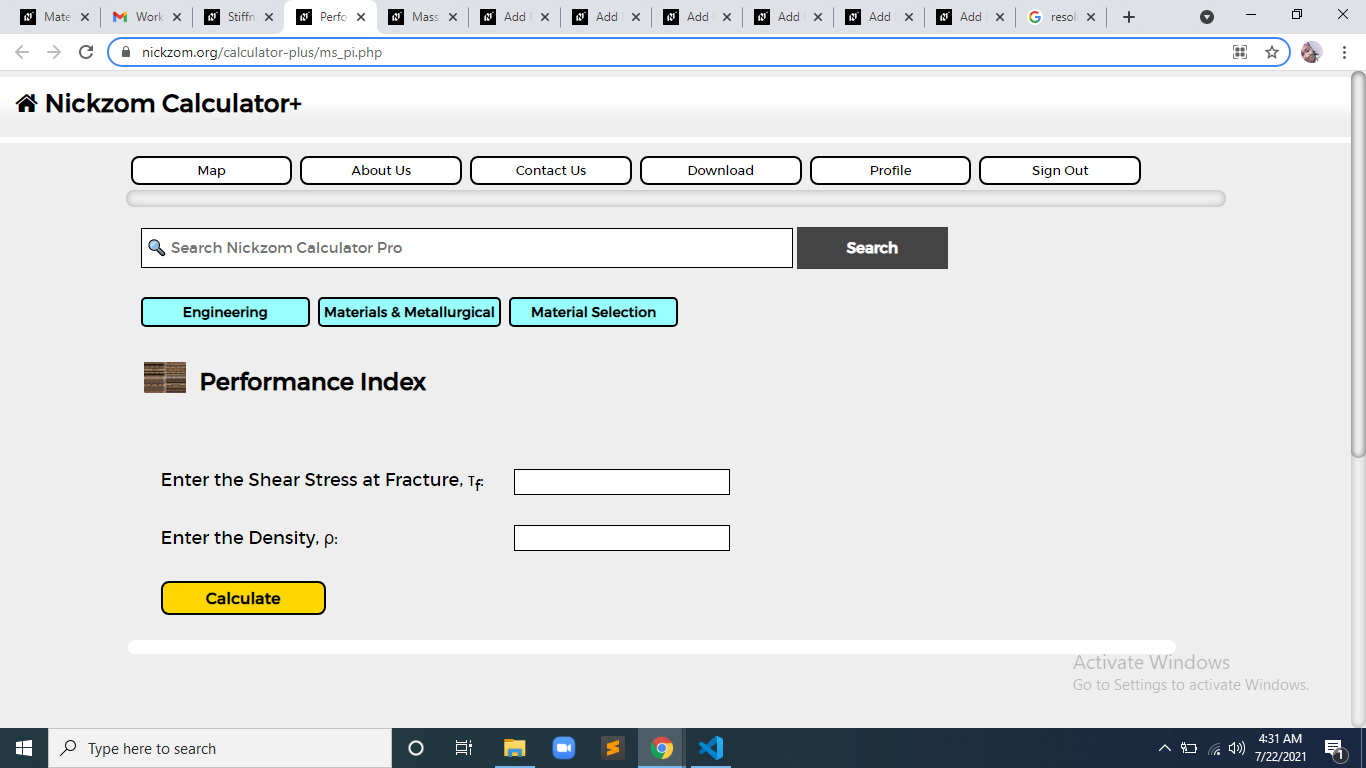Now, enter the values appropriately and accordingly for the parameters as required by the Shear Stress at Fracture (τf) is 32 and Density (ρ) is 10.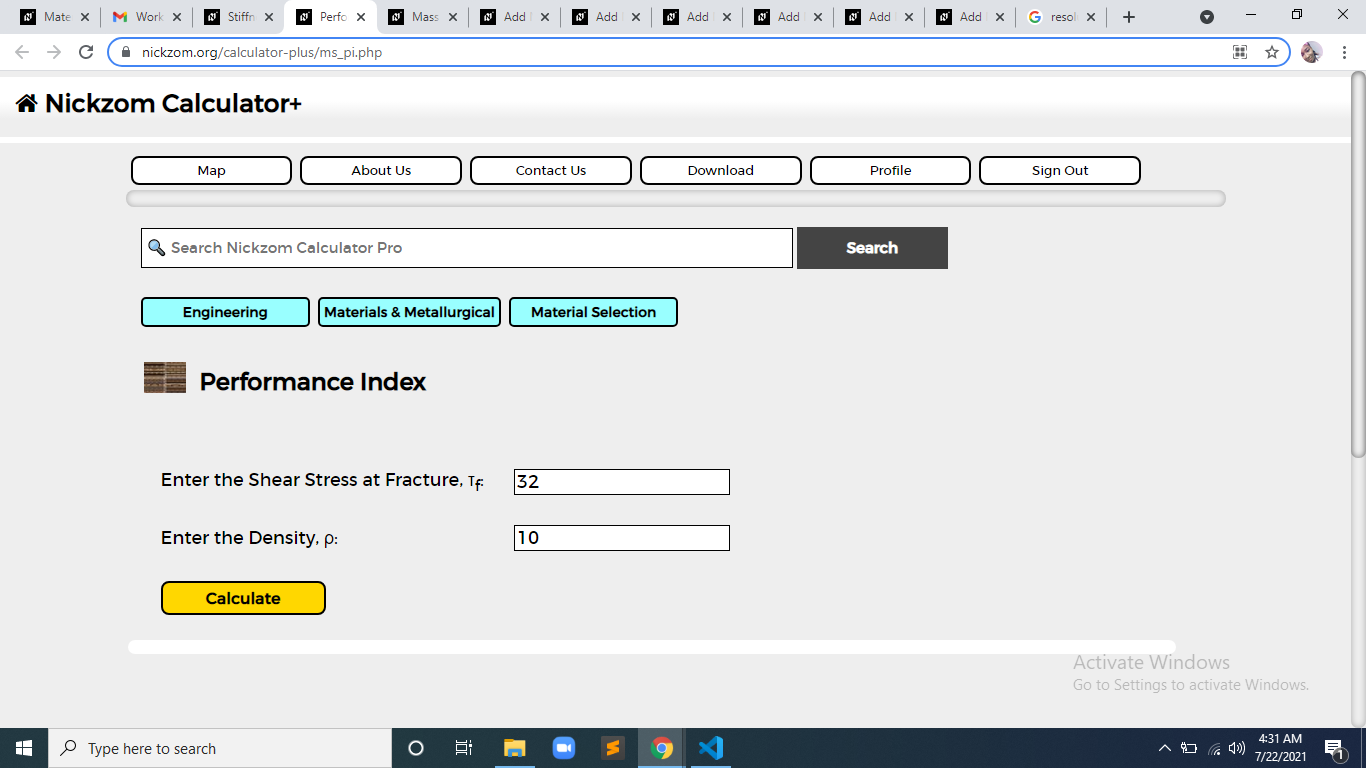Finally, Click on Calculate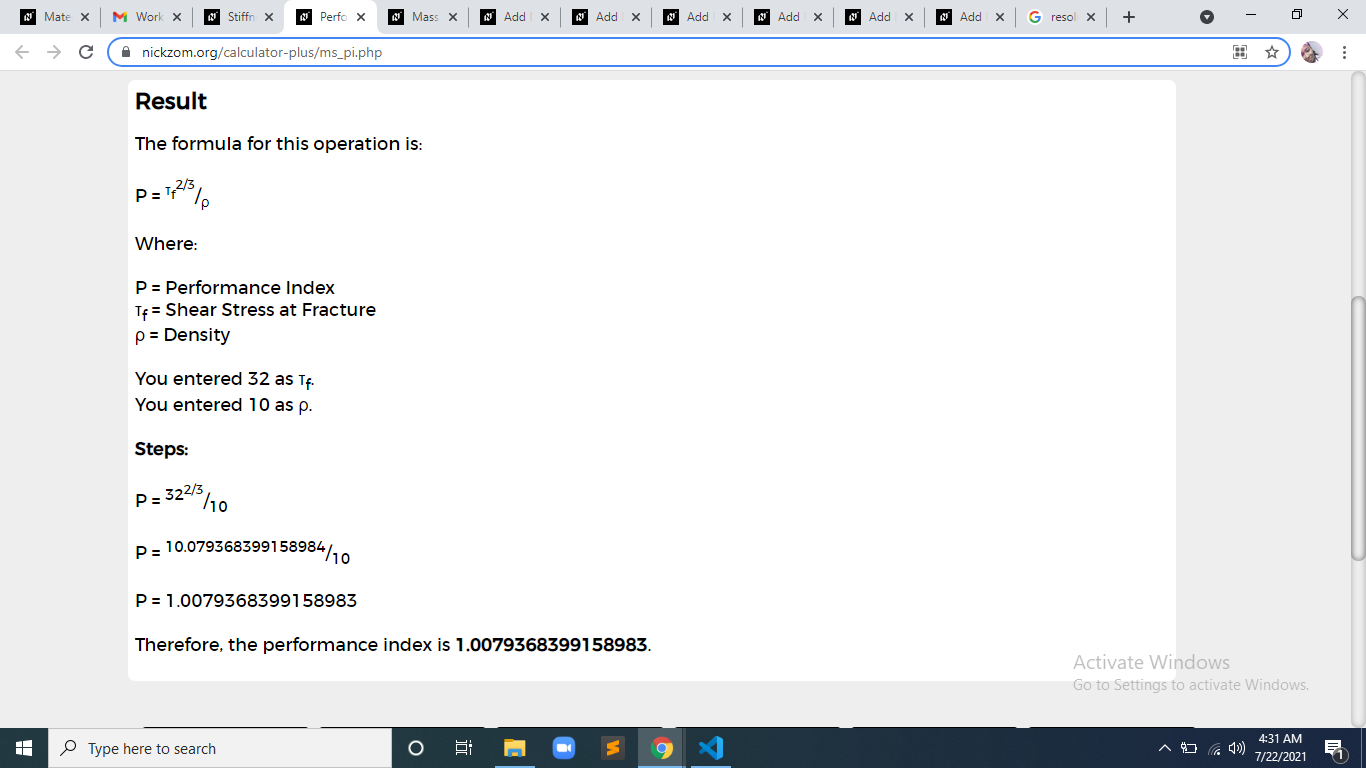As you can see from the screenshot above, Nickzom Calculator– The Calculator Encyclopedia solves for the performance index and presents the formula, workings and steps too.